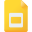# derivative tutorialsDerivatives of Logarithmic Functions
Derivative, High School, United States, Mathematics, Logarithmic FunctionsConcavity and the Second Derivative Test
Applications of Derivatives, High School, United States, Calculus, DifferentiationThe Power Rule and other Rules for Differentiation
Differentiation, High School, United States, Derivative, MathematicsHow Derivatives Affect the Shape of a Graph
Derivative, High School, United States, Differentiation, Applications of Derivatives, Calculus, MathematicsOptimization Problems using Derivatives
Applications of Derivatives, High School, United States, Differentiation, CalculusImplicit Differentiation
Differentiation, High School, United States, Derivative, MathematicsDerivative of a Function
Derivative, High School, United States, Differential Equations, MathematicsProduct Rule and Quotient Rule
Differential Equations, High School, United States, Derivative, MathematicsIncreasing and Decreasing Functions and the First Derivative Test
Derivative, High School, United States, Calculus, Applications of DerivativesDerivatives of Trigonometric Functions
Trigonometric Functions, High School, DerivativeRates of Change in the Natural and Social Sciences
Differentiation, High School, DerivativeThe Chain Rule
Derivative, High School, United States, Chain Rule, MathematicsIndeterminate Forms and L’Hospital’s Rule
Applications of Derivatives, High School, United States, Differentiation, CalculusThe Mean Value Theorem
Derivative, High School, United States, MathematicsLinear Approximations and Differentials with examples
Derivative, High School, United States, Approximations, Calculus, LinearizationHyperbolic Functions and Their Derivatives
Hyperbolic Functions, High School, United States, DerivativeFinding Maxima and Minima using Derivatives
Differentiation, High School, United States, Calculus, DerivativeCurve Sketching using Calculus
Applications of Derivatives, High School, United States, Mathematics, Calculus, Curve SketchingSolving real life problems using differentiation
Exponential Functions, High School, United States, Derivative, Differentiation, CalculusNewton's Method to solve high degree equations
Applications of Derivatives, High School, United States, Calculus, Newton's MethodDiffertitation Rules: Related Rates - Problems and Examples
Derivative, High School, United States, Calculus, Related Rates